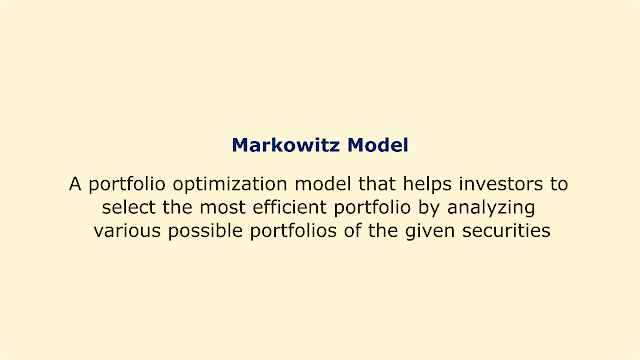# Markowitz ModelImage: Moneybestpal.com

### The Markowitz model is a portfolio optimization tool that assists investors in choosing the most effective portfolio by examining many potential portfolios containing the specified securities.

The model, which is based on the assets' expected returns (mean) and standard deviation (variance), demonstrates how to lower risk by selecting securities that do not move perfectly in lockstep. Harry Markowitz created the model, which is regarded as the cornerstone of contemporary portfolio theory, in 1952.

The main goal of the Markowitz model is to determine the best risk-return trade-off for a portfolio. Return is determined by the mean or expected value of the portfolio returns, but risk is determined by the variance or volatility of those returns. The model implies that investors have a concave and growing utility function, are risk averse, and want higher returns with reduced risk.

To apply the Markowitz model, we need to know the expected return, standard deviation, and correlation of each asset in the portfolio, as well as the risk-free rate. The best weights for each asset can then be determined using mathematical methods like quadratic programming or matrix algebra in order to maximize portfolio return for a given level of risk or decrease portfolio risk for a given level of return. The efficient frontier is the collection of all efficient portfolios that lie on the edge of the feasible zone. Each investor's ideal portfolio is based on their utility function and risk tolerance.

An example of applying the Markowitz model is to construct a portfolio of two assets: stocks and bonds. Assume that the expected return on stocks is 10%, the expected standard deviation is 20%, and the expected return on bonds is 5%, the expected standard deviation is 10%. Bonds and stocks have a 0.2 correlation, and the risk-free rate is 2%. We may calculate the ideal stock and bond weights that maximize portfolio return for various degrees of risk using the Markowitz model.

For instance, if we want a portfolio with a standard deviation of 15%, we can discover that the ideal weights are 50% for stocks and 50% for bonds, and the expected return is 7.5%. If we want a better return of 8%, we must increase the weight of stocks to 60% and decrease the weight of bonds to 40%, but doing so raises the risk to 16.4%. On the other hand, if we want a lower risk of 12%, we must reduce the weight of stocks to 40% and increase the weight of bonds to 60%, but this also reduces the return to 7%.